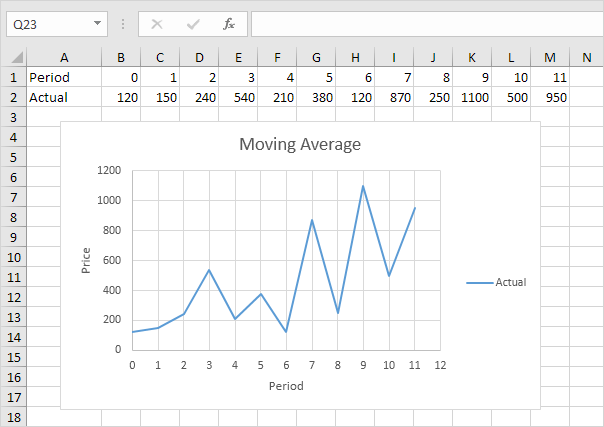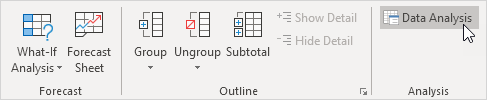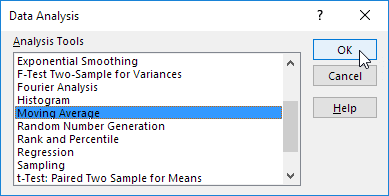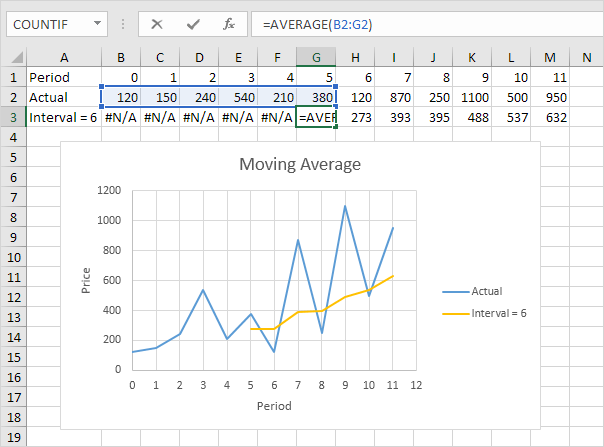# Moving Average

This example teaches you how to calculate the moving average of a time series in Excel. A moving average is used to smooth out irregularities (peaks and valleys) to easily recognize trends.

1. First, let's take a look at our time series.2. On the Data tab, in the Analysis group, click Data Analysis.3. Select Moving Average and click OK.4. Click in the Input Range box and select the range B2:M2.

5. Click in the Interval box and type 6.

6. Click in the Output Range box and select cell B3.

7. Click OK.8. Plot a graph of these values.Explanation: because we set the interval to 6, the moving average is the average of the previous 5 data points and the current data point. As a result, peaks and valleys are smoothed out. The graph shows an increasing trend. Excel cannot calculate the moving average for the first 5 data points because there are not enough previous data points.

9. Repeat steps 2 to 8 for interval = 2 and interval = 4.Conclusion: The larger the interval, the more the peaks and valleys are smoothed out. The smaller the interval, the closer the moving averages are to the actual data points.

Go to Next Chapter: Create a Macro